# Image Approximation with Orthogonal Bases¶

Important: Please read the installation page for details about how to install the toolboxes. $\newcommand{\dotp}{\langle #1, #2 \rangle}$ $\newcommand{\enscond}{\lbrace #1, #2 \rbrace}$ $\newcommand{\pd}{ \frac{ \partial #1}{\partial #2} }$ $\newcommand{\umin}{\underset{#1}{\min}\;}$ $\newcommand{\umax}{\underset{#1}{\max}\;}$ $\newcommand{\umin}{\underset{#1}{\min}\;}$ $\newcommand{\uargmin}{\underset{#1}{argmin}\;}$ $\newcommand{\norm}{\|#1\|}$ $\newcommand{\abs}{\left|#1\right|}$ $\newcommand{\choice}{ \left\{ \begin{array}{l} #1 \end{array} \right. }$ $\newcommand{\pa}{\left(#1\right)}$ $\newcommand{\diag}{{diag}\left( #1 \right)}$ $\newcommand{\qandq}{\quad\text{and}\quad}$ $\newcommand{\qwhereq}{\quad\text{where}\quad}$ $\newcommand{\qifq}{ \quad \text{if} \quad }$ $\newcommand{\qarrq}{ \quad \Longrightarrow \quad }$ $\newcommand{\ZZ}{\mathbb{Z}}$ $\newcommand{\CC}{\mathbb{C}}$ $\newcommand{\RR}{\mathbb{R}}$ $\newcommand{\EE}{\mathbb{E}}$ $\newcommand{\Zz}{\mathcal{Z}}$ $\newcommand{\Ww}{\mathcal{W}}$ $\newcommand{\Vv}{\mathcal{V}}$ $\newcommand{\Nn}{\mathcal{N}}$ $\newcommand{\NN}{\mathcal{N}}$ $\newcommand{\Hh}{\mathcal{H}}$ $\newcommand{\Bb}{\mathcal{B}}$ $\newcommand{\Ee}{\mathcal{E}}$ $\newcommand{\Cc}{\mathcal{C}}$ $\newcommand{\Gg}{\mathcal{G}}$ $\newcommand{\Ss}{\mathcal{S}}$ $\newcommand{\Pp}{\mathcal{P}}$ $\newcommand{\Ff}{\mathcal{F}}$ $\newcommand{\Xx}{\mathcal{X}}$ $\newcommand{\Mm}{\mathcal{M}}$ $\newcommand{\Ii}{\mathcal{I}}$ $\newcommand{\Dd}{\mathcal{D}}$ $\newcommand{\Ll}{\mathcal{L}}$ $\newcommand{\Tt}{\mathcal{T}}$ $\newcommand{\si}{\sigma}$ $\newcommand{\al}{\alpha}$ $\newcommand{\la}{\lambda}$ $\newcommand{\ga}{\gamma}$ $\newcommand{\Ga}{\Gamma}$ $\newcommand{\La}{\Lambda}$ $\newcommand{\si}{\sigma}$ $\newcommand{\Si}{\Sigma}$ $\newcommand{\be}{\beta}$ $\newcommand{\de}{\delta}$ $\newcommand{\De}{\Delta}$ $\newcommand{\phi}{\varphi}$ $\newcommand{\th}{\theta}$ $\newcommand{\om}{\omega}$ $\newcommand{\Om}{\Omega}$

This numerical tour uses several orthogonal bases to perform non-linear image approximation.

In :
from __future__ import division

import numpy as np
import scipy as scp
import pylab as pyl
import matplotlib.pyplot as plt

from nt_toolbox.general import *
from nt_toolbox.signal import *

import warnings
warnings.filterwarnings('ignore')

%matplotlib inline


## Best $M$-terms Non-linear Approximation¶

This tours makes use of an orthogonal base $\Bb = \{ \psi_m \}_{m=0}^{N-1}$ of the space $\RR^N$ of the images with $N$ pixels.

The best $M$-term approximation of $f$ is obtained by a non-linear thresholding

$$f_M = \sum_{ \abs{\dotp{f}{\psi_m}}>T } \dotp{f}{\psi_m} \psi_m,$$

where the value of $T>0$ should be carefully selected so that only $M$ coefficients are not thresholded, i.e.

$$\abs{ \enscond{m}{ \abs{\dotp{f}{\psi_m}}>T } } = M.$$

The goal is to use an ortho-basis $\Bb$ so that the error $\norm{f-f_M}$ decays as fast as possible when $M$ increases, for a large class of images.

This tour studies several different orthogonal bases: Fourier, wavelets (which is at the heart of JPEG-2000), cosine, local cosine (which is at the heart of JPEG).

First we load an image of $N = n \times n$ pixels.

In :
n = 512


Display it.

In :
plt.figure(figsize = (5,5))
imageplot(f)## Fourier Approximation¶

The discrete 2-D Fourier atoms are defined as: $$\psi_m(x) = \frac{1}{\sqrt{N}} e^{ \frac{2i\pi}{n} ( x_1 m_1 + x_2 m_2 ) },$$ where $0 \leq m_1,m_2 < n$ indexes the frequency.

The set of inner products $\{ \dotp{f}{\psi_m} \}_m$ is computed in $O(N \log(N))$ operations with the 2-D Fast Fourier Transform (FFT) algorithm (the pylab function is fft2).

Compute the Fourier transform using the FFT algorithm. Note the normalization by $1/\sqrt{N}$ to ensure orthogonality (energy conservation) of the transform.

In :
fF = pyl.fft2(f)/n


Display its magnitude (in log scale). We use the pylab function fftshift to put the low frequency in the center.

In :
plt.figure(figsize = (5,5))
imageplot(np.log(1e-5 + abs(pyl.fftshift(fF))))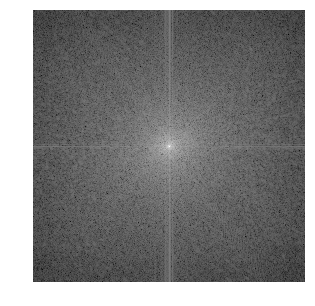An image is recovered from a set of coefficients $c_m$ using the inverse Fourier Transform (pylab function ifft2)) that implements the formula

$$f_M = \sum_m c_m \psi_m.$$

Perform a thresholding.

In :
T = .3
c = np.multiply(fF,(abs(fF) > T))


Inverse the Fourier transform.

In :
fM = np.real(pyl.ifft2(c)*n)


Display the approximation.

In :
plt.figure(figsize = (5,5))
imageplot(clamp(fM))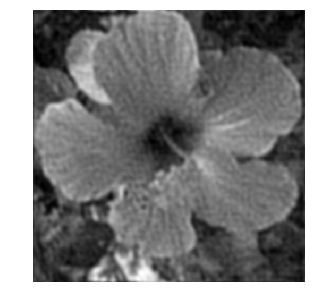Exercise 1

Compute a best $M$-term approximation in the Fourier basis of $f$, for $M \in \{N/100, N/20\}$. Compute the approximation using a well chosen hard threshold value $T$.

In :
run -i nt_solutions/coding_1_approximation/exo1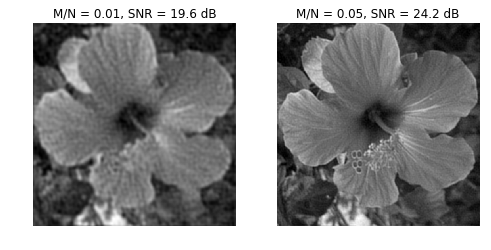In :
## Insert your code here.


The best $M$-term approximation error is computed using the conservation of energy as

$$\epsilon[M]^2 = \norm{f-f_M}^2 = \sum_{ \abs{\dotp{f}{\psi_m}} \leq T } \abs{\dotp{f}{\psi_m}}^2.$$

If one denotes by $\{ c_R[k] \}_{k=0}^{N-1}$ the set of coefficients magnitudes $\abs{\dotp{f}{\psi_m}}$ ordered by decaying magnitudes, then this error is easily computed as $$\epsilon[M]^2 = \sum_{k=M}^{N-1} c_R[k]^2 = \norm{f}^2 - \sum_{k=0}^{M-1} c_R[k]^2.$$ This means that $\epsilon^2$ is equal to $\norm{f}^2$ minus the discrete primitive of $c_R^2$.

Exercise 2

Compute and display in log scales the ordered coefficients $c_R$. Hint: a discrete primitive can be computed using the numpy function cumsum.

In :
run -i nt_solutions/coding_1_approximation/exo2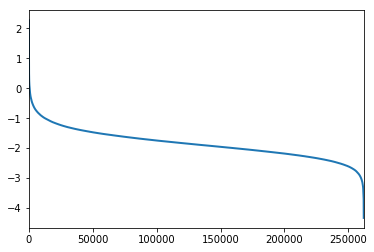In :
## Insert your code here.


Exercise 3

Compute and display in log-scale the non-linear approximation error $\epsilon[M]^2$. Store the values of $\epsilon[M]^2$ in a vector $err\_fft$.

In :
run -i nt_solutions/coding_1_approximation/exo3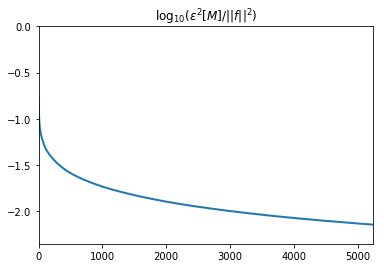In :
## Insert your code here.


## Wavelet Approximation¶

The Wavelet basis of continuous 2-D functions is defined by by scaling and translating three mother atoms $\{\psi^H,\psi^V,\psi^D\}$: $$\psi_{j,n}^k(x) = \frac{1}{2^j}\psi^k\pa{\frac{x-2^j n}{2^j}}$$

Non-linear wavelet approximation is a the heart of the JPEG-2000 compression standard.

The set of inner products $\{ \dotp{f}{\psi_m} \}_m$ is computed in $O(N)$ operations with the 2-D Fast Wavelet Transform algorithm.

Perform a wavelet transform. Here we use a daubechies wavelet transform.

In :
from numpy import linalg
from nt_toolbox.compute_wavelet_filter import *

Jmin = 1
h = compute_wavelet_filter("Daubechies",10)
fW = perform_wavortho_transf(f, Jmin, + 1, h)


Display the coefficients.

In :
plt.figure(figsize = (8,8))

plot_wavelet(fW, Jmin)
plt.title('Wavelet coefficients')

plt.show()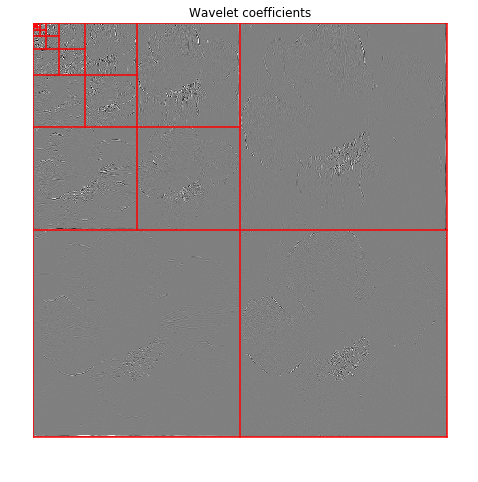Exercise 4

Compute a best $M$-term approximation in the wavelet basis of $f$, for $M \in \{N/100, N/20\}$. Compute the approximation using a well chosen hard threshold value $T$. Note that the inverse wavelet transform is obtained by replacing the +1 by a -1 in the definition of the transform.

In :
run -i nt_solutions/coding_1_approximation/exo4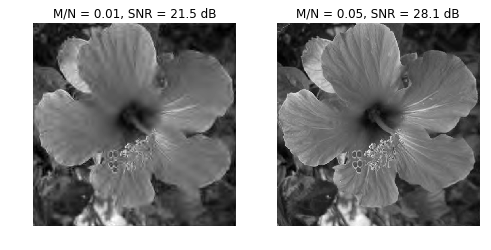In :
## Insert your code here.


Exercise 5

Compute and display in log-scale the non-linear approximation error $\epsilon[M]^2$. Compares the Fourier and wavelets approximations. Store the values of $\epsilon[M]^2$ in a vector $err\_wav$.

In :
run -i nt_solutions/coding_1_approximation/exo5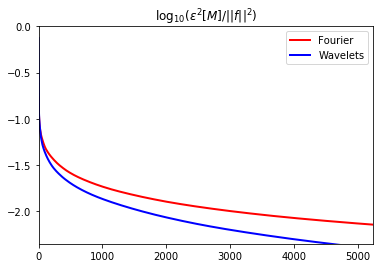In :
## Insert your code here


## Cosine Approximation¶

The discrete cosine approximation (DCT) is similar to the Fourier approximation, excepted that it used symmetric boundary condition instead of periodic boundary condition, and is thus more useful to approximate image.

A 1-D cosine atom of $n$ sample is defined as $$\bar\psi_m(x) = \frac{1}{\sqrt{N}} \cos\pa{ \frac{2\pi}{N} (x-1/2) m }$$ A 2-D cosine atom is obtained by tensor product of 1-D atoms $$\psi_{m_1,m_2}(x_1,x_2) = \bar\psi_{m_1}(x_1) \bar\psi_{m_2}(x_2).$$ On the contrary to the Fourier 2-D atoms, these 2-D DCT atoms are not oriented (they contains 4 Fourier frequencies).

The set of inner products $\{ \dotp{f}{\psi_m} \}_m$ is computed in $O(N \log(N))$ operations with the 2-D Fast Cosine Transform algorithm.

In :
from scipy import fftpack

def dct2(f):
return np.transpose(fftpack.dct(np.transpose(fftpack.dct(f, norm = "ortho")), norm = "ortho"))

def idct2(f):
return np.transpose(fftpack.idct(np.transpose(fftpack.idct(f, norm = "ortho")), norm = "ortho"))

fC = dct2(f)


Display the magnitude of the DCT coefficients. Note that the low frequencies are in the upper-left corner.

In :
plt.figure(figsize = (5,5))
imageplot(np.log(1e-5 + abs(fC)))